# Errata for Multilevel and Longitudinal Modeling Using Stata

The errata for Multilevel and Longitudinal Modeling Using Stata are provided below. Click here for an explanation of how to read an erratum. Click here to learn how to determine the printing number of a book.

 (1) Chapter 1, p. 20, third paragraph, third sentence
 In the top panel, 80% of this variance is due to subjects, whereas in the bottom panel, 80% is due to within-subject variability. In the bottom panel, 80% of this variance is due to subjects, whereas in the top panel, 80% is due to within-subject variability.
 (1) Chapter 1, p. 23, fifth line
 RI Rj
 (1) Chapter 1, p. 29, exercise 1.5.2, line 2
 ... components within and between raters. ... components within and between graders.
 (1) Chapter 1, p. 41, footnote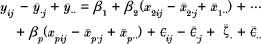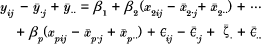(1), (2) Chapter 1, p. 47, second histogram command in the middle of the page
 histogram levm2 if time==1, normal xtitle(Standardized level-2 residuals) histogram lev2m1 if time==1, normal xtitle(Standardized level-2 residuals)
 (1) Chapter 2, p. 54, exercise 2.5, number 3
 Plot mnw versus size, using different symbols for the two treatment groups. Plot mnw versus size, using different symbols for the three treatment groups.
 (1) Chapter 2, p. 54, exercise 2.6, third variable in variable list
 bw birthwt
 (1) Chapter 3, p. 60, commands at the bottom of the page
 . merge school using gcse . sort school . merge school using http://www.stata-press.com/data/mlmus/gcse
 (1), (2) Chapter 3, p. 61, last line
 gcse lrt
 (1) Chapter 3, p. 76, gllamm command line
 . gllamm gcse lrt, i(school) nrf(2) eqs(inter slope) ip(m) nip(15) from(a) copy . gllamm gcse lrt, i(school) nrf(2) eqs(inter slope) ip(m) nip(15) adapt from(a) copy
 (1) Chapter 3, p. 89, gllamm command line
 . gllamm weight age2, nocons i(id) nrf(2) eqs(inter slope) ip(m) nip(15) from(a) geqs(r1 r2) adapt . gllamm weight age2, nocons i(id) nrf(2) eqs(inter slope) ip(m) nip(15) geqs(r1 r2) adapt
 (1) Chapter 3, p. 95, exercise 3.1.4, second sentence
 Also obtain ML (or OLS) estimates of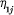and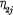by first subtracting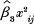with at least two observations. Also obtain ML (or OLS) estimates ofandfirst by subtractingand then by using the statsby command for children with at least two observations.
 (1) Chapter 3, p. 97, exercise 3.4, fourth variable in level-1 variable list
 SES ses
 (1) Chapter 3, p. 98

Switch the order of exercises 3.5.4 and 3.5.5 so that you “fit the random-coefficient model” first.

 (1) Chapter 3, p. 99, exercise 3.6, third description in variable list
 age 14, number of years ... age - 14, number of years ...
 (1) Chapter 3, p. 100, exercise 3.8
 Using (3.2) and (3.3) and the estimates for Model 2 in table 3.1, ... Using (3.2) and the estimates for Model 2 on page 76, ...
 (1) Chapter 4, p. 102, equation 4.1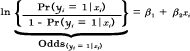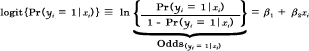(1) Chapter 4, p. 114, last line of Stata command

Replace “Probability” with “Proportion” so that the y-axis title in figure 4.7 is “Proportion of onycholysis“.

 (1) Chapter 4, p. 120, last displayed equation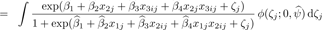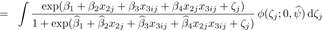(1) Chapter 4, p. 129, fourth equation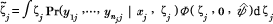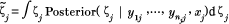(1) Chapter 4, p. 138, exercise 4.5.1, last line
 ... by case (age), sort: gen lag=y[_n-1]. ... by case (age), sort: gen lag = wemp[_n-1].
 (1) Chapter 4, p. 140, exercise 4.7.2, space missing between nr and (year)
 ... by nr(year), sort: gen lag = union[_n-1]. ... by nr (year), sort: gen lag = union[_n-1].
 (1) Chapter 4, p. 142, exercise 4.9.1
 Estimate the values of the estimated school-specific regression ... Guess the values of the estimated school-specific regression ...
 (1) Chapter 5, p. 154, equation (5.2)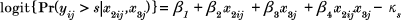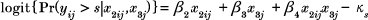(2) Chapter 5, p. 154, equation (5.2)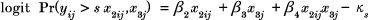(1) Chapter 5, p. 156, table 5.1, estimate of kappa_1 from RC-POM
 -7.31 -7.32
 (1) Chapter 5, p. 158, equation (5.3) and equation in sentence below equation (5.3)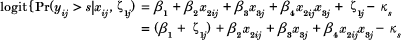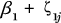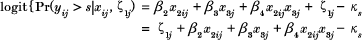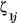(1) Chapter 5, p. 159, equation (5.4)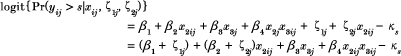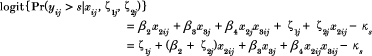(2) Chapter 5, p. 159, equation (5.4)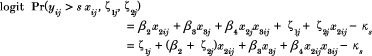(1), (2) Chapter 5, p. 167, section 5.11.1, 3rd equation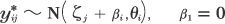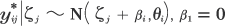(1) Chapter 5, p. 178, exercise 5.4.1, line 3
 ... with a random intercept for graders. ... with a random intercept for essays.
 (2) Chapter 6, p. 182, first equation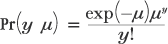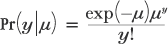(2) Chapter 6, p. 190, chapter heading

#### 6.8 Random-intercept Poisson regression

 (2) Chapter 6, p. 190, chapter subheading
 6. .1 Model specification 6.8.1 Model specification
 (1) Chapter 7, p. 227, table 7.1, log likelihood for model 4
 -349.29 -345.29
 (1) Chapter 7, p. 247, caption for figure 7.5
 (left panel: two-stage formulation ...) (left panel: three-stage formulation ...)
 (1) Chapter 8, p. 261, line 1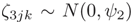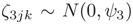(1) References, p. 304 and throughout the book
 Hedeker, D. and R. D. Gibbons. 1996a. Applied Longitudinal Data Analysis. Chichester, UK: Wiley. Hedeker, D. and R. D. Gibbons. Forthcoming. Applied Longitudinal Data Analysis. Chichester, UK: Wiley.